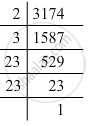Advertisement Remove all ads

# By What Numbers Should Each of the Following Be Divided to Get a Perfect Square? Also, Find the Number Whose Square is the New Number. 3174 - Mathematics

By what numbers should each of the following be divided to get a perfect square? Also, find the number whose square is the new number.

3174

Advertisement Remove all ads

#### Solution

3174 = 2 x 3 x 23 x 23Grouping them into pairs of equal factors:
3174 = 2 x 3 x (23 x 23)
The factors, 2 and 3 are not paired. For a number to be a perfect square, each prime factor has to be paired. Hence, 3174 must be divided by 6 (2 x 3) for it to be a perfect square.
The new number would be (23 x 23).
Hence, the number whose square is the new number is 23.

Is there an error in this question or solution?
Advertisement Remove all ads

#### APPEARS IN

RD Sharma Class 8 Maths
Chapter 3 Squares and Square Roots
Exercise 3.1 | Q 8.4 | Page 4
Advertisement Remove all ads

#### Video TutorialsVIEW ALL 

Advertisement Remove all ads
Share
Notifications

View all notifications

Forgot password?
Course